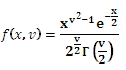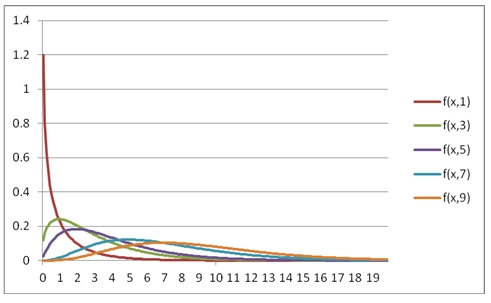# SQL Server CHISQ.DIST function

CHISQPDF

Updated: 31 July 2010

Use CHISQPDF to calculate the probability density function of the chi square distribution.

The probability density function is:Syntax
SELECT [wctStatistics].[wct].[CHISQPDF] (
<@X, float,>
,<@Degrees_freedom, float,>)
Arguments
@X
is any real number. @X is an expression of type float or of a type that implicitly converts to float.
@Degrees_Freedom
is the degrees of freedom. @Degrees_Freedom is an expression of type float or of a type that implicitly converts to float.
Return Types
float
Remarks
·         @DF must be greater than zero (@DF > 0).
Examples
SELECT wct.CHISQPDF(1,3) as [CHISQ pdf]

This produces the following result
CHISQ pdf
----------------------
0.241970724519143
You can use the SeriesFloat function from the XLeratorDB/math library to generate a dataset which can be pasted into EXCEL to generate a graph of the function.
SELECT SeriesValue
,wct.CHISQPDF(SeriesValue, 1) as [f(x,1)]
,wct.CHISQPDF(SeriesValue, 3) as [f(x,3)]
,wct.CHISQPDF(SeriesValue, 4) as [f(x,5)]
,wct.CHISQPDF(SeriesValue, 7) as [f(x,7)]
,wct.CHISQPDF(SeriesValue, 9) as [f(x,9)]
FROM wct.SeriesFloat(0.1,20,.1,NULL,NULL)

This is an EXCEL-generated graph of the results### SupportCopyright 2008-2020 Westclintech LLC         Privacy Policy        Terms of Service